Courses

# Test: Measurement Of Length (2) - 3

## 20 Questions MCQ Test Mathematics for Class 3: NCERT | Test: Measurement Of Length (2) - 3

Description
This mock test of Test: Measurement Of Length (2) - 3 for Class 3 helps you for every Class 3 entrance exam. This contains 20 Multiple Choice Questions for Class 3 Test: Measurement Of Length (2) - 3 (mcq) to study with solutions a complete question bank. The solved questions answers in this Test: Measurement Of Length (2) - 3 quiz give you a good mix of easy questions and tough questions. Class 3 students definitely take this Test: Measurement Of Length (2) - 3 exercise for a better result in the exam. You can find other Test: Measurement Of Length (2) - 3 extra questions, long questions & short questions for Class 3 on EduRev as well by searching above.
QUESTION: 1

Solution:

QUESTION: 2

### The squares below increase in size from left to right. If the pattern continues, how long will each side of the next square be?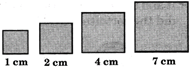Solution:

1 + 1 = 2 cm 2 + 2 = 4 cm 4 + 3 = 7 cm 7 + 4 = 11 cm

QUESTION: 3

### The table below shows how far three students jumped.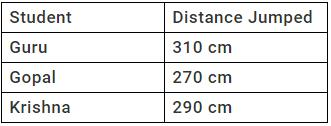Which number sentence can be used to find how many centimetres did Guru jumped than Krishna?

Solution:
QUESTION: 4

Mr. and Mrs. Guru Ride bikes as exercise. The table shows the total number of kilometres they had ridden after different number of days.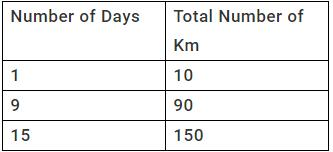If the pattern continues, how many kilometres will Mr. and Mrs. Guru have ridden after 28 days?

Solution:

28 x 10 = 280 km

QUESTION: 5

Heera, Meera and Neera each measured the length of a room using their own feet as measuring tools.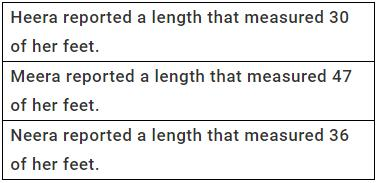Which of the three students had the smallest feet?

Solution:
QUESTION: 6

The drawing shows a shelf that measures 10 metres. It is divided into 5 sections of equal length.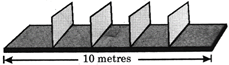What is the length of each section?

Solution:

10 metres ÷ 5 = 2 metres

QUESTION: 7

3 m =   _______ cm

Solution:

1 m = 100 cm 3 m = 3 x 100 cm = 300 cm

QUESTION: 8

A train travels from P to S as shown below: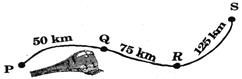What is the total distance from P to S?

Solution:

50 km + 75 km + 125 km = 250 km

QUESTION: 9

8 m 5 cm = ______ cm

Solution:

8 m 5 cm = 800 cm + 5 cm = 805 cm

QUESTION: 10

m: km :: g : ?

Solution:

1000 m = 1 km; Similarly 1000 g = 1 kg.

QUESTION: 11

If the length of the rope needed to make border P is 8 cm, then find the length of rope needed for border Q, if the distance between every two points is same.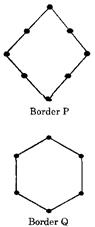Solution:
QUESTION: 12

Which is longest?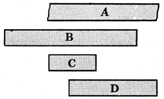Solution:
QUESTION: 13

By what measure is the line A longer than line B?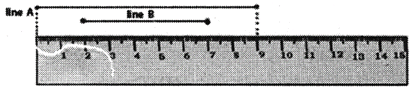Solution:

9 cm ? 5 cm = 4 cm

QUESTION: 14

In a running race, rabbit is 100 m ahead of tortoise. After that it takes rest for sometime. At that time tortoise crosses it and travels 50 m. How much distance did the tortoise travel?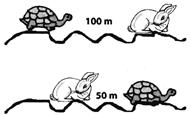Solution:

100 m + 50 m = 150 m

QUESTION: 15

A string of 30 cm long has been cut into 3 equal pieces. What is the length of each piece?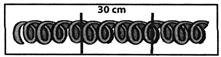Solution:

30 cm ÷ 3 = 10 cm

QUESTION: 16

Sachin hits a ball to boundary and it travels along the dotted line as shown below. How much distance does it cover?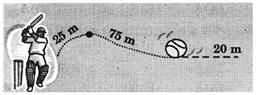Solution:

25 m + 75 m + 20 m = 120 m

QUESTION: 17

Which of the following is different from other three?

Solution:

gm is the unit of mass but other three are units of length.

QUESTION: 18

Choose the odd one from the following measures?

Solution:
QUESTION: 19

In the adjoining rectangular scenery, AB = 2 metres then CD = _________ centimetres.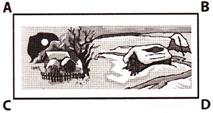Solution:

AB = CD (since in a rectangle opposite sides are equal).
∴ CD = 2 metres = 2 x 100 cm = 200 centimetres

QUESTION: 20

Write the following in ascending order? 2m, 50cm, 100 mm

Solution: